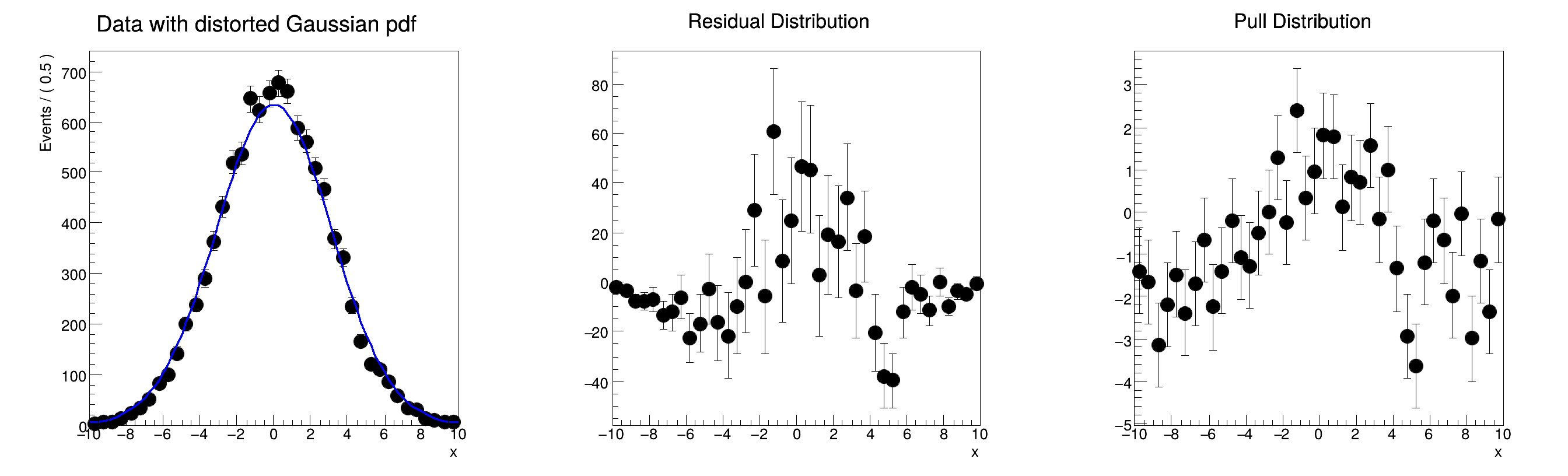ROOT   Reference Guiderf109_chi2residpull.C File Reference

## Detailed DescriptionBasic functionality: Calculating chi^2 from histograms and curves in RooPlots, making histogram of residual and pull distributions[1mRooFit v3.60 -- Developed by Wouter Verkerke and David Kirkby[0m
Copyright (C) 2000-2013 NIKHEF, University of California & Stanford University
chi^2 = 2.63198
#include "RooRealVar.h"
#include "RooDataSet.h"
#include "RooGaussian.h"
#include "RooConstVar.h"
#include "TCanvas.h"
#include "TAxis.h"
#include "RooPlot.h"
#include "RooHist.h"
using namespace RooFit;
void rf109_chi2residpull()
{
// S e t u p m o d e l
// ---------------------
// Create observables
RooRealVar x("x", "x", -10, 10);
// Create Gaussian
RooRealVar sigma("sigma", "sigma", 3, 0.1, 10);
RooRealVar mean("mean", "mean", 0, -10, 10);
RooGaussian gauss("gauss", "gauss", x, RooConst(0), sigma);
// Generate a sample of 1000 events with sigma=3
RooDataSet *data = gauss.generate(x, 10000);
// Change sigma to 3.15
sigma = 3.15;
// P l o t d a t a a n d s l i g h t l y d i s t o r t e d m o d e l
// ---------------------------------------------------------------------------
// Overlay projection of gauss with sigma=3.15 on data with sigma=3.0
RooPlot *frame1 = x.frame(Title("Data with distorted Gaussian pdf"), Bins(40));
gauss.plotOn(frame1);
// C a l c u l a t e c h i ^ 2
// ------------------------------
// Show the chi^2 of the curve w.r.t. the histogram
// If multiple curves or datasets live in the frame you can specify
// the name of the relevant curve and/or dataset in chiSquare()
cout << "chi^2 = " << frame1->chiSquare() << endl;
// S h o w r e s i d u a l a n d p u l l d i s t s
// -------------------------------------------------------
// Construct a histogram with the residuals of the data w.r.t. the curve
RooHist *hresid = frame1->residHist();
// Construct a histogram with the pulls of the data w.r.t the curve
RooHist *hpull = frame1->pullHist();
// Create a new frame to draw the residual distribution and add the distribution to the frame
RooPlot *frame2 = x.frame(Title("Residual Distribution"));
// Create a new frame to draw the pull distribution and add the distribution to the frame
RooPlot *frame3 = x.frame(Title("Pull Distribution"));
TCanvas *c = new TCanvas("rf109_chi2residpull", "rf109_chi2residpull", 900, 300);
c->Divide(3);
c->cd(1);
frame1->GetYaxis()->SetTitleOffset(1.6);
frame1->Draw();
c->cd(2);
frame2->GetYaxis()->SetTitleOffset(1.6);
frame2->Draw();
c->cd(3);
frame3->GetYaxis()->SetTitleOffset(1.6);
frame3->Draw();
}
Date
July 2008

Definition in file rf109_chi2residpull.C.

c
#define c(i)
Definition: RSha256.hxx:101
RooPlot::Draw
virtual void Draw(Option_t *options=0)
Draw this plot and all of the elements it contains.
Definition: RooPlot.cxx:691
RooPlot::residHist
RooHist * residHist(const char *histname=0, const char *pdfname=0, bool normalize=false, bool useAverage=kFALSE) const
Return a RooHist containing the residuals of histogram 'histname' with respect to curve 'curvename'.
Definition: RooPlot.cxx:1136
RooFit::Bins
RooCmdArg Bins(Int_t nbin)
Definition: RooGlobalFunc.cxx:177
RooAbsData::SumW2
@ SumW2
Definition: RooAbsData.h:97
RooFit::DataError
RooCmdArg DataError(Int_t)
Definition: RooGlobalFunc.cxx:157
RooGaussian.h
x
Double_t x[n]
Definition: legend1.C:17
RooGaussian
Plain Gaussian p.d.f.
Definition: RooGaussian.h:24
TCanvas.h
RooDataSet.h
RooPlot::chiSquare
Double_t chiSquare(int nFitParam=0) const
Shortcut for RooPlot::chiSquare(const char* pdfname, const char* histname, int nFitParam=0)
Definition: RooPlot.h:176
void addPlotable(RooPlotable *plotable, Option_t *drawOptions="", Bool_t invisible=kFALSE, Bool_t refreshNorm=kFALSE)
Add the specified plotable object to our plot.
Definition: RooPlot.cxx:570
TGeant4Unit::gauss
static constexpr double gauss
Definition: TGeant4SystemOfUnits.h:263
RooFit
The namespace RooFit contains mostly switches that change the behaviour of functions of PDFs (or othe...
Definition: RooCFunction1Binding.h:29
RooAbsData::plotOn
virtual RooPlot * plotOn(RooPlot *frame, const RooCmdArg &arg1=RooCmdArg::none(), const RooCmdArg &arg2=RooCmdArg::none(), const RooCmdArg &arg3=RooCmdArg::none(), const RooCmdArg &arg4=RooCmdArg::none(), const RooCmdArg &arg5=RooCmdArg::none(), const RooCmdArg &arg6=RooCmdArg::none(), const RooCmdArg &arg7=RooCmdArg::none(), const RooCmdArg &arg8=RooCmdArg::none()) const
Definition: RooAbsData.cxx:547
RooPlot.h
RooPlot::GetYaxis
TAxis * GetYaxis() const
Definition: RooPlot.cxx:1258
RooPlot
A RooPlot is a plot frame and a container for graphics objects within that frame.
Definition: RooPlot.h:44
RooRealVar.h
RooHist
A RooHist is a graphical representation of binned data based on the TGraphAsymmErrors class.
Definition: RooHist.h:27
RooHist.h
RooConstVar.h
RooPlot::pullHist
RooHist * pullHist(const char *histname=0, const char *pdfname=0, bool useAverage=false) const
Uses residHist() and sets normalize=true.
Definition: RooPlot.h:181
sigma
const Double_t sigma
Definition: h1analysisProxy.h:11
TCanvas
The Canvas class.
Definition: TCanvas.h:23
TAxis.h
RooDataSet
RooDataSet is a container class to hold unbinned data.
Definition: RooDataSet.h:33
TAttAxis::SetTitleOffset
virtual void SetTitleOffset(Float_t offset=1)
Set distance between the axis and the axis title.
Definition: TAttAxis.cxx:293
RooRealVar
RooRealVar represents a variable that can be changed from the outside.
Definition: RooRealVar.h:37
RooFit::Title
RooCmdArg Title(const char *name)
Definition: RooGlobalFunc.cxx:176
RooFit::RooConst
RooConstVar & RooConst(Double_t val)
Definition: RooGlobalFunc.cxx:347